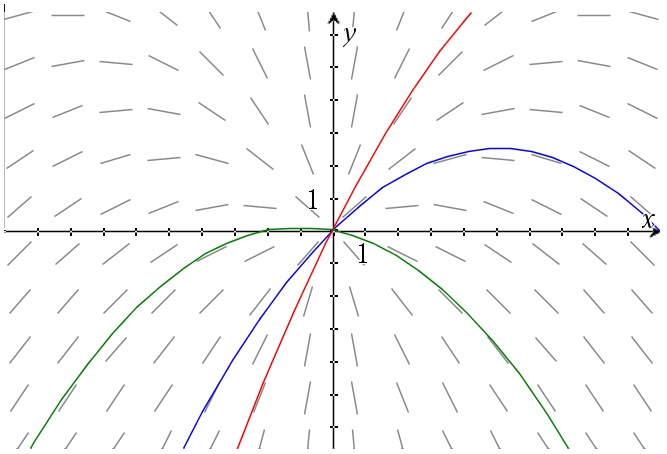# First order linear differential equations – a couple of examples

Up a level : Differential Equations
Previous page : First order linear differential equations
Next page : Using Taylor series to solve differential equationsExample 1

Let us solve$y' - xy = x$

This is actually a separable equation, but let us first solve it as linear differential equation. We have p(x)= –x so that gives us$I = {e^{\int {( - x)dx} }} = {e^{ - \frac{{{x^2}}}{2}}}$

Multiplying our equation by this gives us${e^{ - \frac{{{x^2}}}{2}}}y' - {e^{ - \frac{{{x^2}}}{2}}}xy = {e^{ - \frac{{{x^2}}}{2}}}x$

or${\left( {{e^{ - \frac{{{x^2}}}{2}}}y} \right)^\prime } = {e^{ - \frac{{{x^2}}}{2}}}x$

So${e^{ - \frac{{{x^2}}}{2}}}y = \int {{e^{ - \frac{{{x^2}}}{2}}}xdx}$

If we now do the variable substitution$\left| \begin{gathered} w = - \frac{{{x^2}}}{2} \hfill \\ - dw = xdx \hfill \\ \end{gathered} \right.$

We get${e^{ - \frac{{{x^2}}}{2}}}y = - \int {{e^w}dw = {e^w} + C = - } {e^{ - \frac{{{x^2}}}{2}}} + C$

Multiplying away the first factor, we get$y = {e^{\frac{{{x^2}}}{2}}}( - {e^{ - \frac{{{x^2}}}{2}}} + C) = C{e^{\frac{{{x^2}}}{2}}} - 1$

that is our final solution. Interestingly enough it is the same solutions as we found to$\frac{{dy}}{{dx}} = xy$

but just shifted down one unit. As we can see we could write$y' - xy = x$

as$y' = x + xy$

or$y' = x(1 + y)$

so as mentioned it is actually separable. We get$\frac{{dy}}{{y + 1}} = xdx$

or$\ln |y + 1| = \frac{{{x^2}}}{2} + {C_1}$

and thus$y + 1 = C{e^{\frac{{{x^2}}}{2}}}$

and finally$y = C{e^{\frac{{{x^2}}}{2}}} - 1$

just as expected.

Example 2

Let us take an old example and modify it slightly. If the slopes where directed out from the origin we had that$\frac{{dy}}{{dx}} = \frac{y}{x}$

and that gave us solutions of the form$y = Cx$

I.e. straight lines through the origin. Now let us change that a bit. Say we are subtracting or adding) a bit from the slope, and that what we subtract is proportional to the distance from the y-axis. Say we have$\frac{{dy}}{{dx}} = \frac{y}{x} - 0.1x$

This can be written as$y' - \frac{y}{x} = - 0.1x$

So it is a linear first order equation with$p(x) = - \frac{1}{x}$

This gives us$I = {e^{ - \int {\frac{{dx}}{x}dx} }} = {e^{ - \ln x}} = {e^{\ln {x^{ - 1}}}} = \frac{1}{x}$

Multiplying through with this gives us$\frac{{y'}}{x} - \frac{y}{{{x^2}}} = - 0.1$

or${\left( {\frac{{y'}}{x}} \right)^\prime } = - 0.1$

Integration now gives us$\frac{{y}}{x} = - 0.1x + C$

that gives us$y = - 0.1{x^2} + Cx$

This looks rather pleasing as a graph, with a few particular solutions.As you can see all the solutions will be parabolas passing through the origin.

To find particular solutions passing through a particular point (x0,y0) we just substitute in those values into our solution to get${y_0} = - 0.1x_0^2 + C{x_0}$

or$C = \frac{{{y_0} + 0.1x_0^2}}{{{x_0}}}$

This gives us the particular solution$C = \frac{{{y_0} + 0.1x_0^2}}{{{x_0}}}$Up a level : Differential Equations
Previous page : First order linear differential equations
Next page : Using Taylor series to solve differential equationsLast modified: Dec 26, 2020 @ 15:13